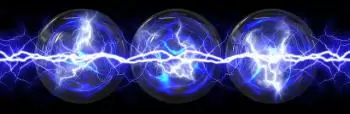# What Is Electric Potential Energy?

In physics, electrical potential energy is the potential energy of an electrostatic field. This means that if a point electric charge is placed at a point, it will exert a conservative force on any other charge present. In this case, the potential energy of a charge depends on the set of charges that it has around it.Electric potential energy is also called electrostatic potential energy when we refer to systems with constant electric fields over time.

This concept is similar to that of gravitational potential energy. In gravitational potential energy, an object at a certain height is affected by an external force that draws it towards the center of the earth. When it comes to electrical potential energy, a charged body subjected to an electrical force acquires electrical potential energy (Ep).

## Definition of Electric Potential Energy

The electric potential energy of a system of two charges placed at a distance between them is defined as the work done by the electric force when the two charges are brought an infinite distance between them.

Basically it corresponds to the energy that a charge possesses by the mere fact of being within an electric field at a certain distance from the source charge and indicates the potential kinetic energy that the charge can express if it is left free to move.

## Work Done to Shift an Electrical Charge

The work done by the electric force to move an electric charge from point A to point B causes the charges to acquire potential energy. If we release it, the force that pulls them away will convert the potential energy into work.

The work to move a particle from point A to point B in an electric field is the difference of the potential energies at these two points. This means that the work these fields do to move an electric charge between two points in space does not depend on the path followed but only on the initial and final position.

## What Is the Formula for Electric Potential Energy?

The electric potential energy that has a point charge q in the presence of another point charge Q separated between them is:

Ep = K⋅Q⋅q / r

where:

• Ep represents electric potential energy. In the international system of measurements it is measured in Joules (J).

• Q and q are the values ​​of the two point charges expressed in Coulombs (C).

• r is the distance that separates them expressed in meters (m).

• K is the constant of Coulomb's law. For vacuum its value is approximately 9 · 10 ^ 9 N · m ^ 2 / C ^ 2.

If the charges have the same sign, the electric potential energy is positive, if there is a positive and a negative charge, the potential energy will be negative.

## What Is the Electric Potential?

The potential energy per unit charge is called the electric potential. This concept is very general since the potential at a point is independent of the test load

Author:

Published: September 7, 2021
Last review: September 7, 2021